Fractions as part of a whole number

Whole numbers are called integers.

Examples of whole numbers are 2, 3, 7, 21 and 341.

A fraction is a part of an integer or a whole number. The image below tries to define a fraction as being a portion of a whole number. "a fraction of a the whole"A Fraction  is actually the quotient ratio form of two quantities.

Video on fractions

A fraction is expressed as:

1. Numerator
2. Denominator

#### Simplifying fractions by cancellation

A fraction can be simplified or reduced to a value when its numerator and denominator have no common factors.

Working with the fraction is easier when they're simplified. We work with fractions when we multiply, subtract add or divide.

An equivalent fraction where the numerator and denominator have no common factors is used to simplify the fraction.

1. Make a list the prime factors of the numerator and denominator.
2. Identify the prime factors common to both the numerator and denominator.
3. Use cancelling to divide the numerator and denominator by all common factors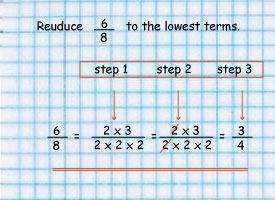#### Second Example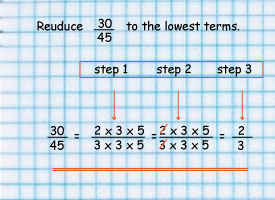Simplify `56/64`

``

Simplify`24/36`

## Types of fractions

1. Proper fractions: where the numerator is less the denominator for example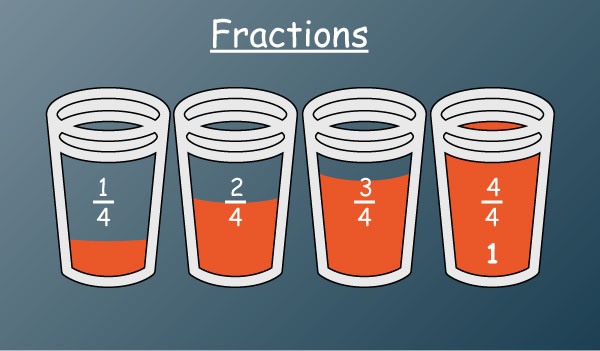2. Improper fractions: where the numerator is greater than the denominator for example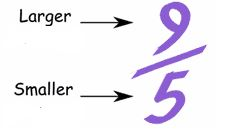3Mixed number fractions as called mixed numbers: consists of a whole number and a proper fraction e.g.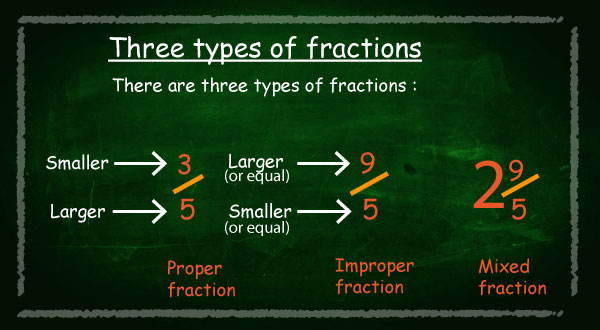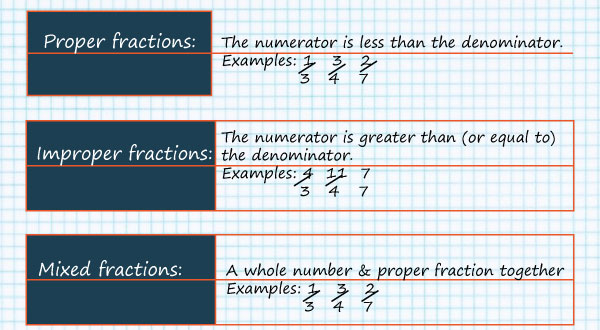[resource: 977, align: left]

### Mixed fractions to improper fractions

To convert mixed fractions to improper fractions follow the following steps;

Step 1: multiply the denominator with the whole number

Step 2: add the numerator to the product of step 1 to get the new numerator

Step 3: The denominator of the  remains the same.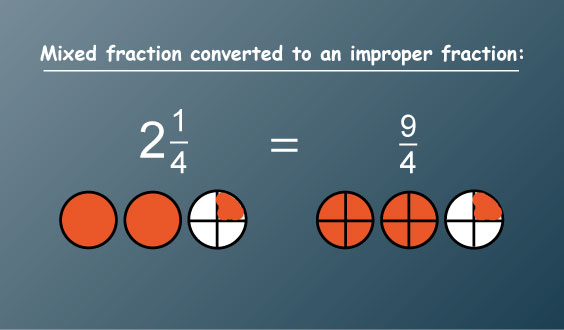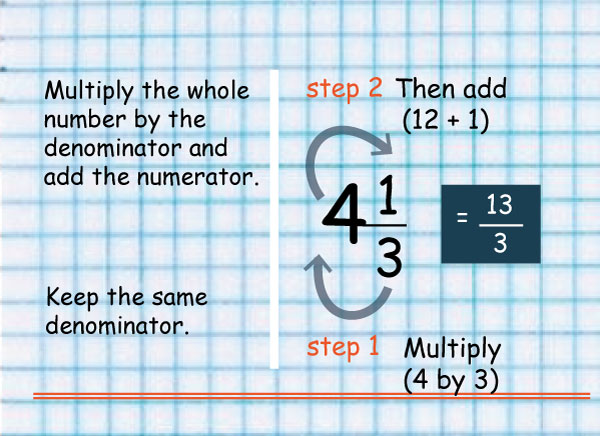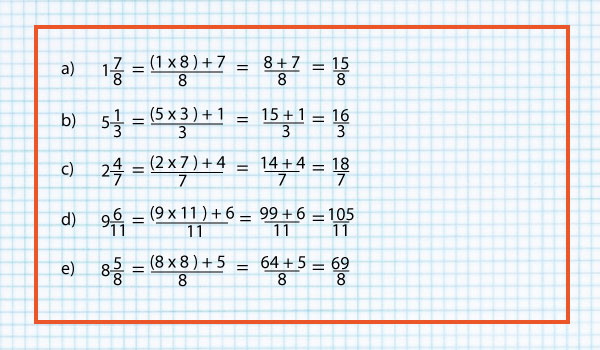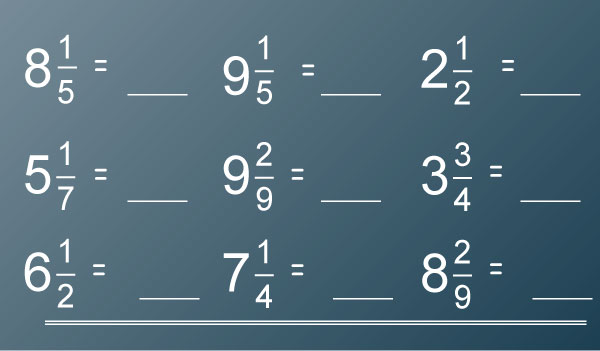### Improper Fractions to Mixed fractions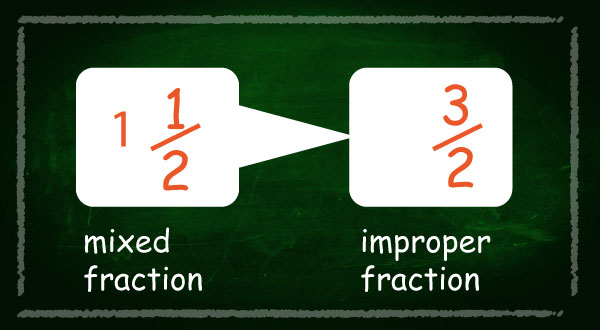To convert improper fractions to mixed fractions follow the steps below;

Step 1: divide the numerator with the denominator,

Step 2: add the quotient is whole number and the remainder is the new numerator while the denominator remains the same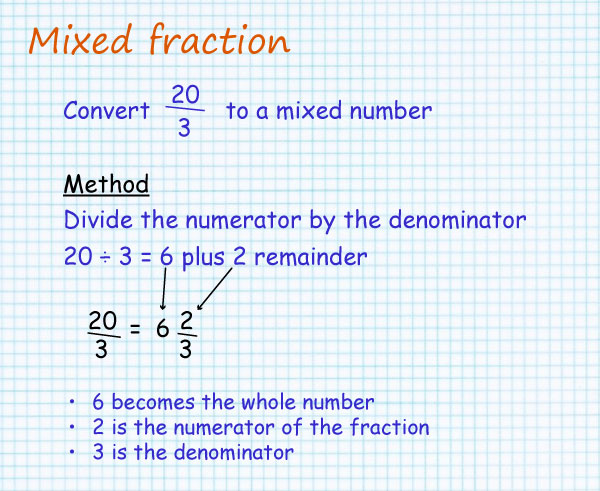### Sources

• FasPart-Img1 by elimu used under CC_BY-SA
• intro_to_fractions_1 by elimu used under CC_BY-SA
• FasPart-Img3 by

elimu

used under CC_BY-SA
• FasPart-Img4 by elimu used under CC_BY-SA
• fractions_improper by

elimu

used under CC_BY-SA
• fractions_part_of_a_whole_1 by

elimu

used under CC_BY-SA
• fractions_simplify_by_cancellation_1 by

elimu

used under CC_BY-SA
• fractions_simplify_by_cancellation_3 by

elimu

used under CC_BY-SA
• M2.1.2 by

elimu

used under CC_BY-SA
• page_2.1.2 by

elimu

used under CC_BY-SA
• FasPart-Img2 by

elimu

used under CC_BY-SA
• FasPart-Img7 by

elimu

used under CC_BY-SA
• FasPart-Img8 by

elimu

used under CC_BY-SA
• FasPart-Img9 by

elimu

used under CC_BY-SA
• fractions_improper_1 by

elimu

used under CC_BY-SA
• FasPart-Img10 by

elimu

used under CC_BY-SA
• FasPart-Img11 by

elimu

used under CC_BY-SA
• FasPart-Img12 by

elimu

used under CC_BY-SA
• FasPart-Img13 by

elimu

used under CC_BY-SA
• FasPart-Img14 by

elimu

used under CC_BY-SA
• FasPart-Img15 by

elimu

used under CC_BY-SA
• FasPart-Img16 by

elimu

used under CC_BY-SA

•